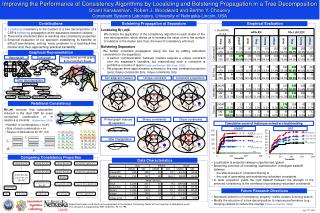DownloadDownload PresentationContributions

# Contributions

Download Presentation## Contributions

- - - - - - - - - - - - - - - - - - - - - - - - - - - E N D - - - - - - - - - - - - - - - - - - - - - - - - - - -
##### Presentation Transcript

1. Improving the Performance of Consistency Algorithms by Localizing and Bolstering Propagation in a Tree Decomposition ShantKarakashian, Robert J. Woodward and Berthe Y. Choueiry Constraint Systems Laboratory, University of Nebraska-Lincoln, USA C1 {A,B,C,N},{R1} E C7 C2 {A,I,N},{} {B,C,D,H},{R6} R2 R1 R3 R1 C3 C4 C8 A,B,C,N N B {I,M,N},{R2} {A,I,K},{} {B,D,F,H},{} R4 C10 A C R2 R5 B A D C C6 C9 C5 D C,D,H B,D,E,F A,K,L I,M,N {I,J,K},{R3} {F,G,H},{R7} {A,K,L},{R4} {B,D,E,F},{R5} R6 K H F R3’ R6 R5 R7 I R4 C1 I,J,K F,G,H A D B C C7 R3 R7 • Contributions • Bolstering Propagation at Separators • Empirical Evaluation C N C2 C8 C3 E E E E B D • Localizing consistency to the clusters of a tree decomposition of a CSP & bolstering propagation at the separators between clusters • Theoretical characterization of resulting new consistency properties • Empirical evaluation of our approach establishing its benefits on difficult benchmarks, solving many problems in a backtrack-free manner and, thus, approaching ‘practical tractability’ Localizing R(∗,m)C R2 R1 R3 A R2 R1 R3 R2 R1 R3 R2 R1 R3 I M Ry C5 We localize the application of the consistency algorithm to each cluster of the tree decomposition, which allows us to increase the value of m to the number of relations in the cluster and, thus, the level of consistency enforced. Ra R4 K F H B A D C B A D C B A D C B A D C Rsep R4 C4 L J Rx C6 R6 R5 R7 R6 R5 R7 R7 R6 R5 R7 R6 R5 R’3 R’3 C9 C10 E G F F F Bolstering Separators • We bolster constraint propagation along the tree by adding redundant constraints to the separators. • A perfect ‘communication’ between clusters requires a unique constraint over the separator’s variables, but materializing such a constraint is prohibitive in terms of space [Fattah and Dechter 1996; Kask+ 2005]. • We propose three approximation schemes to this end: constraint projection (proj), binary constraints (bin), clique constraints (clq). • Graphical Representation • Hypergraph • Dual graph • Triangulated primal graph & its maximal cliques R1 N A B C R2 R4 D R6 R5 I J K E R3 M L H G F ..… R7 • Two adjacent clusters • Unique constraint • Constraint projections • Tree decomposition Ri R3 R4 R7 φ R2 R1 R5 F • Relational Consistency R6 R(∗,m) ensures that subproblem induced in the dual CSP by every connected combination of m relations is minimal [Karakshian+ 2010] • Primal graph induced @ separator… • Binary constraints • Clique constraints Cumulative count of instances solved w/o backtracking • Number of combinations = O(em) • Size of each combination = m • Twelve combinations for R(*,3)C F • UNSAT • SAT • {R1,R2,R3} • {R1,R2,R4} • {R1,R2,R5} • {R1,R2,R6} • {R1,R3,R4} • {R1,R4,R5} • {R1,R4,R6} • {R1,R5,R6} • {R1,R5,R7} • {R1,R6,R7} • {R2,R3,R4} • {R5,R6,R7} • cl-proj-R(∗,|ψ(cli)|)C • cl-proj-R(∗,|ψ(cli)|)C • cl-proj-wR(∗,3)C • cl-R(∗,|ψ(cli)|)C • … after triangulation • cl-proj-wR(∗,2)C • GAC • cl-proj-wR(∗,3)C • cl-R(∗,|ψ(cli)|)C • cl-proj-wR(∗,2)C • GAC • Comparing Consistency Properties • Data Characteristics τj • Localization & projection always outperformed ‘global’ • Bolstering schemes of increasing ‘sophistication’ investigate tradeoff between • the effectiveness of constraint filtering & • the cost of generating and maintaining redundant constraints • In tests, projection yields the right tradeoff between the strength of the enforced consistency & the overhead of processing redundant constraints τi cl+clq-R(∗,2)C cl+clq-R(∗,3)C cl+clq-R(∗,4)C cl+clq-R(∗,|ψ(cli)|)C cl+bin-R(∗,3)C cl+bin-R(∗,4)C cl+bin-R(∗,|ψ(cli)|)C cl+bin-R(∗,2)C cl+proj-R(∗,2)C R(∗,2)C R(∗,4)C cl+proj-R(∗,3)C R(∗,3)C cl+proj-R(∗,4)C cl+proj-R(∗,|ψ(cli)|)C maxRPWC A D B C GAC cl-R(∗,2)C cl-R(∗,3)C cl-R(∗,4)C cl-R(∗,|ψ(cli)|)C • Future Research Directions • Automatic selection of consistency property: inside clusters & during search • Modify the structure of a tree decomposition to improve performance (e.g., merging clusters to reduce the overlap[Fattah and Dechter 1996]) Experiments were conducted on the equipment of the Holland Computing Center at the University of Nebraska-Lincoln. This research is supported by NSF Grant No. RI-111795. July 17th, 2013